Online Tution   »   Class 10   »   Pair Of Linear Equations In Two...

# Pair Of Linear Equations In Two Variables Class 10 Notes Ch-3

## Pair Of Linear Equations In Two Variables Class 10 Notes

A linear equation is a straight-line equation. It takes the shape of

ax + by + c = 0

Where a, b and c are the real numbers (a≠0 and b≠0) and x and y are the two variables, Here, a and b are the coefficients, and c is the equation’s constant.

## Pair Of Linear Equations in two variable

Two Linear Equations having two same variables are the pair of Linear Equations in two variables.

• a1x + b1y + c1 = 0
• a2x + b2y + c2 = 0

## Pair Of Linear Equations In Two Variables Class 10- Graphical Method Of Solution

There will be two lines on the graph because we are displaying two equations.

1. 1. If the two lines cross at that specific position, there will only be one solution to the pair of linear equations. It is claimed that the two equations are compatible.

1. There will be an endless number of answers if the two lines meet, as every point along the line will contain the answer to the pair of linear equations. It is referred to as a pair of dependent or consistent equations.

1. There won’t be a solution if the two lines are parallel because they don’t connect anywhere. It is claimed that the equations are incoherent.

## Pair Of Linear Equations In Two Variables Class 10- Algebraic Methods Of Solving

These procedures must be followed in order to solve a pair of linear equations with the variables x and y using the substitution method:

### Substitution Method

Step 1: Pick any equation and determine the value of one variable in relation to another, in this case, y in relation to x.

Step 2: Next, change the other equation to use the estimated value of y in terms of x.

Step 3: Since there is only one variable in this linear equation, x, we must now solve it in terms of x.

Step 4: Change the value of x in the provided equations and determine the value of y.

Using this approach, we can eliminate any one of the variables to solve the equations.

Ace your class 10th board exams with Adda247 live classes for class 10th preparation.

### Elimination Method

Step 1: Multiply the coefficients of each variable in both equations by a certain number to make them equal.

Step 2: Now combine or divide the equations so that one variable, whose coefficients are the same, is eliminated.

Step 3: To determine the value of the residual variable, solve the equation.

Step 4: Use the equations provided to obtain the value of the other variable by substituting the calculated value of the variable.

### Cross Multiplication Method

Given two equations in the form of

a1x + b1y + c1 = 0 and a2x + b2y + c2 = 0, where

We write it in general form as

To apply cross multiplication, we use this diagram

The multiplied pairs are shown by the arrows. The product of the upward and downward arrow pair products must be subtracted from one another.

Using this diagram, we must first write the equations in general form; then, we must calculate the values of x and y and enter those values in the corresponding notations.

## Equations Reducible To A Pair Of Linear Equations In two variables

In this section, we learn about such equations, which are not linear but can be reduced to pair of linear equations form using the substitution method. Let us understand with the help of an example.

2/x+3/y=4

5/x−4/y=9

In this case, we may make the substitution

1/x = u and 1/y = v

The pair of equations reduces to

2u + 3v = 4 …..(i)

5u – 4v = 9 ….(ii)

From the first equation, isolate the value of u.

u = (4-3v)/2

Now substitute the value of u in eq. (ii)

5[(4-3v)/2] – 4v = 9

Solving for v, we get;

v = 2/23

Now substitute the value of v in u = (4-3v)/2 to get the value of u.

u = 43/23

Since, u = 1/x or x = 1/u = 23/43

and v = 1/y or y = 1/v, so y = 23/2

Hence, the solutions are x = 23/43 and y = 23/2.

Let’s connect via chat or call our senior expert counsellor at +91-9625869989 to learn more about the different streams and options available.

We would love it if we could add some of your insights. If you have a definite goal of scoring the highest marks, then you can resolve your doubts via our app/quizzes and youtube class  assistance (https://youtube.com/c/Adda247Class9and10)

Related Post:

Polynomials Class 10 Chapter 2

## Pair Of Linear Equations In Two Variables Class 10- FAQs

Ques. A linear equation has how many potential solutions?

Ans. The graphs of a system of linear equations have an infinite number of solutions.

Ques. Consistency of a dependent pair of linear equations is it guaranteed?

Ans. Since coincidental lines are consistent and intersecting lines are also consistent, the dependent pair of linear equations is always true. This is due to the fact that the dependent pair of equations is also known as coincidental lines or merely coincidental equations.

Ques. How many solutions may there be for parallel lines?

Ans. There are no solutions when the lines are parallel, and there are a finite number of solutions when the two equations graph the same line.

Ques. What does it mean when an equation has an answer of 0?

Ans. When you find the solution to the two equations, they must equal 0=0 for the answer to having an infinite solution.

Ques. How can you know if there isn’t a solution to an equation?

Ans. The problem has no solutions if you attempt to solve an equation with false results.

Ques. How many different solutions exist for a pair of linear equations?

Ans. Three algebraic strategies can be used to resolve a pair of linear equations in two variables.

Ques. A two-variable linear equation has how many possible solutions?

Ans. A linear equation with two variables also has an endless number of solutions.

Sharing is caring!

Thank You, Your details have been submitted we will get back to you.
•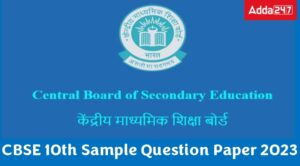CBSE Class 10 Sample Papers 2022-23 for ...
•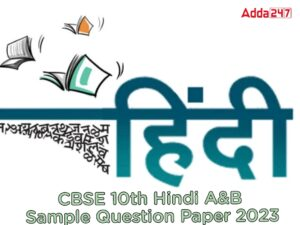CBSE Class 10 Hindi A & B Sample Pap...
•CBSE Class 12 Maths Sample Paper 2022-23...
•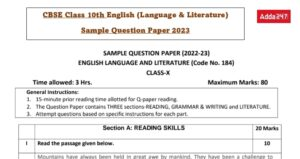Class 10 English Sample Paper 2022- 23 w...
•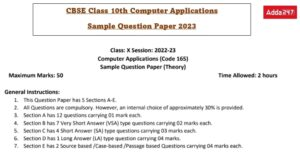CBSE Class 10 Computer Applications Samp...
•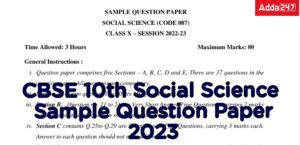Class 10 Social Science (SST) Sample Pap...